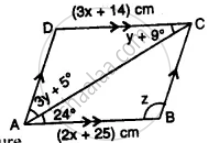# Use the Information Given in the Alongside Diagram to Find the Value of X, Y and Z. - Mathematics

Sum

Use the information given in the alongside diagram to find the value of x, y, and z.#### Solution

ABCD is a parallelogram and AC is its diagonal which bisects the opposite angle

Opposite sides of a parallelogram are equal

3x + 14 = 2x + 25

⇒ 3x – 2x = 25 – 14

⇒ x = 11

∴ x = 11 cm

∠DCA = ∠CAB (Alternate angles)

y + 9° = 24

y = 24° – 9° = 15°

∠DAB = 3y° + 5° + 24° = 3 x 15 + 5 + 24° = 50° + 24° = 74°

∠ABC =180°- ∠DAB = 180° – 74° = 106°

z = 106°

Hence x = 11 cm, y = 15°, z = 106°

Concept: Types of Quadrilaterals - Properties of a Parallelogram
Is there an error in this question or solution?

#### APPEARS IN

Selina Concise Mathematics Class 8 ICSE
Chapter 17 Special Types of Quadrilaterals
Exercise 17 | Q 20 | Page 199